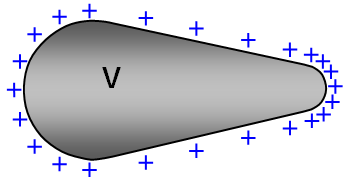# Electric charge distribution – concentration at points

Question: Why does the electric charge distribute itself unevenly on a conductor's surface? Why does charge concentrate at a point on a conductor? For example on a lightning conductor.

Charge distribution over a surface.
1. We must assume that the potential is equal over the surface. This will be true when equilibrium is reached – a very short time after the conducting solid has been charged.
2. Let the charge density of a surface be denoted by σ
3. The potential at a distance r from a sphere carrying a charge Q is given by:

Potential (V) = [1/4πεo]Q/r

4. Charge density of the sphere (s) = Q/4πr2
5. Potential (V) = [1/4πεo]σ4πr2/r = [1/εo]σr and so Charge density (σ) = Vεo/r
6. Therefore the charge density at a point on the surface of radius r is inversely proportional to r. In other words a small r (a sharply curved surface) has a greater charge density than a surface with large R.Think of two spheres of different radii charged to the same potential. If they are joined together by a wire no charge will flow between them (same potential) but the charge density on each will be different. Now instead of a wire fit a charged conducting surface (same potential as the spheres) between them to form the shape shown in the following figure. Again there will be no charge flow and the charge distribution will therefore be different at different points on the surface.

(Note that the lines of the electric field will always be at right angles to the surface).

WORD VERSION AVAILABLE ON THE SCHOOLPHYSICS CD